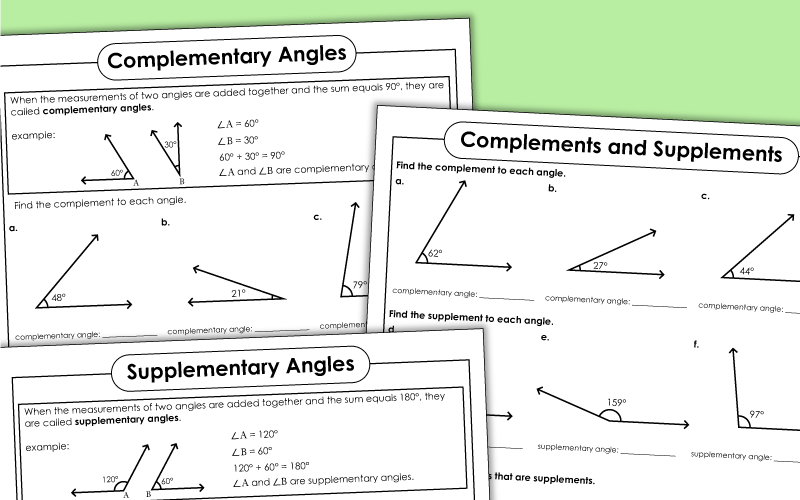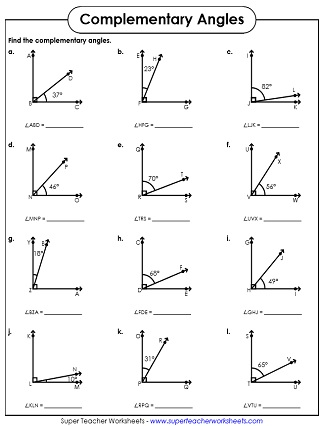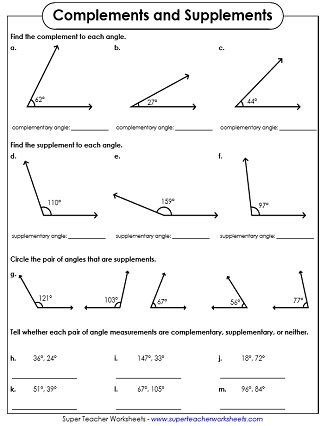# Complementary, Supplementary, & Vertical Angles

The geometry worksheets on this page can be used to introduce and review the concepts of complementary, supplementary angles, and vertical angles.## Complementary & Supplementary Angles

Students find complements and supplements. Then they circle a pair of angles that are supplements. Then they determine whether each pair of angles is complementary, supplementary, or neither.
Find the complements and supplements of the angles shown on the cards. This file includes 30 cards that can be used for learning centers, peer help sessions, or on your document camera.

## Complementary Angles

Pairs of complementary angles are shown. The measurement of one is given. Students must calculate the measurement of the other.
This worksheet serves as a basic introduction to complementary angles. At the top, the concept is described. At the bottom, students are instructed to find the complement to each angle shown.
Cut out the angle tiles at the bottom of the page. Glue each angle next to it next to its complement.

## Supplementary Angles

On each problem, students are shown two angles. The measurement of one angle is known. The other is the supplement, which must be calculated.
This is a very basic introduction to supplementary angles. At the top of the page is an explanation box. Then students are shown nine angles. They need to find the supplement for each.
Cut out the angle picture squares at the bottom of the worksheet. Then glue each one next to its supplement.

This page has illustrations of 2 adjacent angles. Students must recognize the combined angle as additive. There's also an angle word problem that requires students to divide.

## Complementary, Supplementary, & Vertical Angles

Identify complementary, supplementary, and vertical angles. Calculate the number of degrees in each unknown angle.
This page has information about complementary, supplementary, and vertical angles, with examples. At the bottom, students identify the angle type and calculate the unknown measurements.
Circle the correct type of angle and find the unknown measurement. There are 12 problems on this worksheet.
Angle Types and Measuring Angles

Here you'll find a collection of printables for teaching students how to measure angles with a protractor. There are also worksheets on types of angles (obtuse, acute, right).

Area of Triangles

Surface Area

Here you'll find a selection of worksheets for teaching surface area.

Geometry Worksheet Index

Here's our geometry index page that will link you to all geometry topics on our site.

## Sample Worksheet ImagesMy Account
Site Information